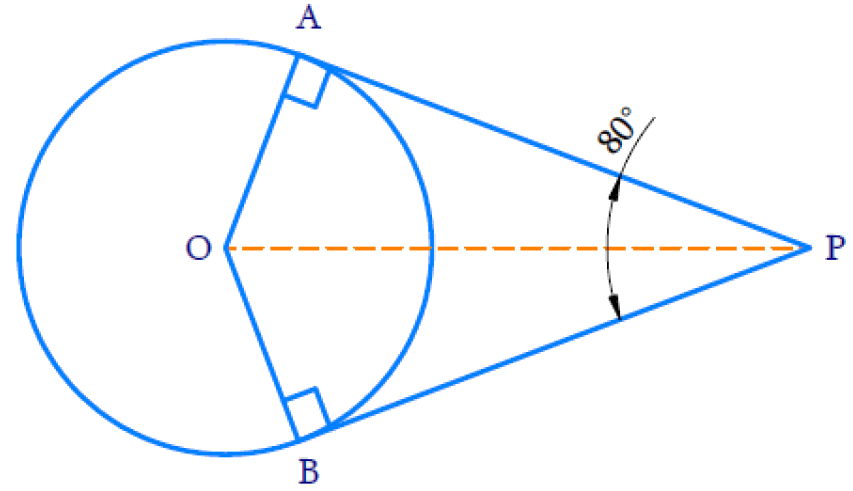# Ex.10.2 Q3 Circles Solution - NCERT Maths Class 10

Go back to  'Ex.10.2'

## Question

If tangents $$PA$$ and $$PB$$ from a point $$P$$ to a circle with center $$O$$ are inclined to each other at angle of $$80^\circ,$$ then $$\angle {POA}$$ is equal to

(A)$$50^\circ$$

(B)$$60^\circ$$

(C)$$70^\circ$$

(D)$$80^\circ$$

Video Solution
Circles
Ex 10.2 | Question 3

## Text Solution

What is Known?

$$PA$$ and $$PB$$ are the tangents from $$P$$ to a circle with center $$O.$$ Tangents are inclined to each other at angle of $$80^\circ$$What is Unknown?

$$\angle {POA}$$

Reasoning:

• The lengths of tangents drawn from an external point to a circle are equal
• Tangent at any point of a circle is perpendicular to theradius through the point of contact.

Steps:

In $$\triangle {OAP}$$ and in $$\triangle {OBP}$$

$$OA = OB$$ (radii of the circle are always equal)

$$AP = BP$$ (length of the tangents)

$$OP = OP$$ (common)

Therefore, by $$SSS$$ congruency $$\Delta {OAP} \cong \Delta {O B P}$$

SSS congruence rule: If three sides of one triangle are equal to the three sides of another triangle, then the two triangles are congruent.

If two triangles are congruent then their corrresponding pars are equal.

Hence,

$$\angle POA = \angle POB \\\angle OPA = \angle OPB$$

Therefore, $$OP$$ is the angle bisector of $$\angle {APB}$$ and $$\angle {AOB}$$

\begin{align} \therefore \quad \angle {O P A}&= \angle O P B\\& = \frac { 1 } { 2 } ( \angle A P B ) \\ & = \frac { 1 } { 2 } ( 80 ) \\ & = 40 ^ { \circ } \end{align}

By angle sum property of a triangle, In $$\Delta {OAP}$$

$\angle {A} + \angle {POA} + \angle {OPA} = 180 ^ { \circ }$

From the figure,

$${OA} \, \bot \, {AP}$$ (Theorem 10.1: The tangent at any point of a circle is perpendicular to the radius through the point of contact.)

\begin{align} \therefore \quad \angle {A} &= 90 ^ { \circ } \\ 90 ^ { \circ } + \angle { POA } + 40 ^ { \circ } &= 180 ^ { \circ } \\ 130 ^ { \circ } + \angle { POA }& = 180 ^ { \circ } \\ \angle { POA } & = 180 ^ { \circ } - 130 ^ { \circ } \\ \angle { POA } & = 50 ^ { \circ } \end{align}

The correct Option is A

Learn from the best math teachers and top your exams

• Live one on one classroom and doubt clearing
• Practice worksheets in and after class for conceptual clarity
• Personalized curriculum to keep up with school